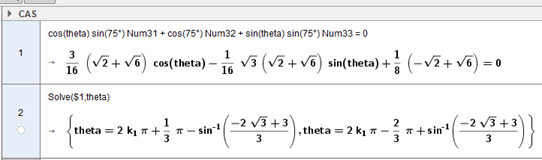# Calculate points on sphere?

SharkD shared this question 2 years ago

In this workbook:

Could someone please tell me how to calculate the coordinates of the intersection points of the latitude and longitude curves with the outermost circumcircle? I do not know the formulas.

Thank you!

Mike1

`Polar coordinates of point Q can be obtained using abs(Q) and arg(Q) (and also alt(Q) for 3D points).`1

I don't understand? They are plotted cartesian 2D points not polar or 3D points.1

I mean the actual plotted points, not the figure being represented.1

I have attached a better example maybe.

For example if:

`cos(theta) sin(75°) Num31 + cos(75°) Num32 + sin(theta) sin(75°) Num33 = 0`

How do I determine theta? (Two possible answers.)21

I don't know how to type that into GeoGebra. When I type it I get:

`cos(t h ℯ t a) sin(75°) Num31 + cos(75°) Num32 + sin(t h ℯ t a) sin(75°) Num33 = 0`
Where h and a are checkboxes that evaluate to true. (Are you looking at my second example/attachment?)1

Never mind. The CAS interface works differently.

But the solutions I am getting don't make sense. Do I need to convert the solution to degrees or is it automatic?1

The result by default is in radians, so I had to take that into account later on.

The problem now is that the CAS command creates a list, but if I try to access an item in that list I get an "Illegal Argument" error. (See attachment.) The list itself does render the result as points if made visible.1

I am looking for an alternative that does not live inside CAS view.

I plotted the function

`s = cos(x) sin(75°) Num31 + cos(75°) Num32 + sin(x) sin(75°) Num33`

Then I created the intersection points of this function and the x axis. Unfortunately, these points shift right or left along the function over time as I rotate the sphere using the sliders. Sometimes they are a large distance away. Sometimes they occupy the same location. I need them to stay somewhat in the same place relative to the function and to each other.

Can I set min and max extents of the function?11

I have attached the file.

See points A and B when you move slider yaw_1.